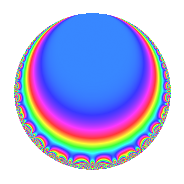# Properties

 Label 804.2.o.aLevel 804 Weight 2 Character orbit 804.o Analytic conductor 6.420 Analytic rank 1 Dimension 2 CM disc. -3 Inner twists 4

# Related objects

## Newspace parameters

 Level: $$N$$ = $$804 = 2^{2} \cdot 3 \cdot 67$$ Weight: $$k$$ = $$2$$ Character orbit: $$[\chi]$$ = 804.o (of order $$6$$ and degree $$2$$)

## Newform invariants

 Self dual: No Analytic conductor: $$6.41997232251$$ Analytic rank: $$1$$ Dimension: $$2$$ Coefficient field: $$\Q(\sqrt{-3})$$ Coefficient ring: $$\Z[a_1, \ldots, a_{13}]$$ Coefficient ring index: $$1$$ Sato-Tate group: $\mathrm{U}(1)[D_{6}]$

## $q$-expansion

Coefficients of the $$q$$-expansion are expressed in terms of a primitive root of unity $$\zeta_{6}$$. We also show the integral $$q$$-expansion of the trace form.

 $$f(q)$$ $$=$$ $$q + ( -1 + 2 \zeta_{6} ) q^{3} + ( -4 + 2 \zeta_{6} ) q^{7} -3 q^{9} +O(q^{10})$$ $$q + ( -1 + 2 \zeta_{6} ) q^{3} + ( -4 + 2 \zeta_{6} ) q^{7} -3 q^{9} + ( -3 - 3 \zeta_{6} ) q^{13} + ( 8 - 8 \zeta_{6} ) q^{19} -6 \zeta_{6} q^{21} -5 q^{25} + ( 3 - 6 \zeta_{6} ) q^{27} + ( 2 - \zeta_{6} ) q^{31} + ( -10 + 10 \zeta_{6} ) q^{37} + ( 9 - 9 \zeta_{6} ) q^{39} + ( -1 + 2 \zeta_{6} ) q^{43} + ( 5 - 5 \zeta_{6} ) q^{49} + ( 8 + 8 \zeta_{6} ) q^{57} + ( -9 - 9 \zeta_{6} ) q^{61} + ( 12 - 6 \zeta_{6} ) q^{63} + ( -2 - 7 \zeta_{6} ) q^{67} + ( -7 + 7 \zeta_{6} ) q^{73} + ( 5 - 10 \zeta_{6} ) q^{75} + ( -14 + 7 \zeta_{6} ) q^{79} + 9 q^{81} + 18 q^{91} + 3 \zeta_{6} q^{93} + ( -11 - 11 \zeta_{6} ) q^{97} +O(q^{100})$$ $$\operatorname{Tr}(f)(q)$$ $$=$$ $$2q - 6q^{7} - 6q^{9} + O(q^{10})$$ $$2q - 6q^{7} - 6q^{9} - 9q^{13} + 8q^{19} - 6q^{21} - 10q^{25} + 3q^{31} - 10q^{37} + 9q^{39} + 5q^{49} + 24q^{57} - 27q^{61} + 18q^{63} - 11q^{67} - 7q^{73} - 21q^{79} + 18q^{81} + 36q^{91} + 3q^{93} - 33q^{97} + O(q^{100})$$

## Character Values

We give the values of $$\chi$$ on generators for $$\left(\mathbb{Z}/804\mathbb{Z}\right)^\times$$.

 $$n$$ $$269$$ $$337$$ $$403$$ $$\chi(n)$$ $$-1$$ $$\zeta_{6}$$ $$1$$

## Embeddings

For each embedding $$\iota_m$$ of the coefficient field, the values $$\iota_m(a_n)$$ are shown below.

For more information on an embedded modular form you can click on its label.

Label $$\iota_m(\nu)$$ $$a_{2}$$ $$a_{3}$$ $$a_{4}$$ $$a_{5}$$ $$a_{6}$$ $$a_{7}$$ $$a_{8}$$ $$a_{9}$$ $$a_{10}$$
365.1
 0.5 − 0.866025i 0.5 + 0.866025i
0 1.73205i 0 0 0 −3.00000 1.73205i 0 −3.00000 0
641.1 0 1.73205i 0 0 0 −3.00000 + 1.73205i 0 −3.00000 0
 $$n$$: e.g. 2-40 or 990-1000 Significant digits: Format: Complex embeddings Normalized embeddings Satake parameters Satake angles

## Inner twists

Char. orbit Parity Mult. Self Twist Proved
1.a Even 1 trivial yes
3.b Odd 1 CM by $$\Q(\sqrt{-3})$$ yes
67.d Odd 1 yes
201.f Even 1 yes

## Hecke kernels

This newform can be constructed as the kernel of the linear operator $$T_{5}$$ acting on $$S_{2}^{\mathrm{new}}(804, [\chi])$$.